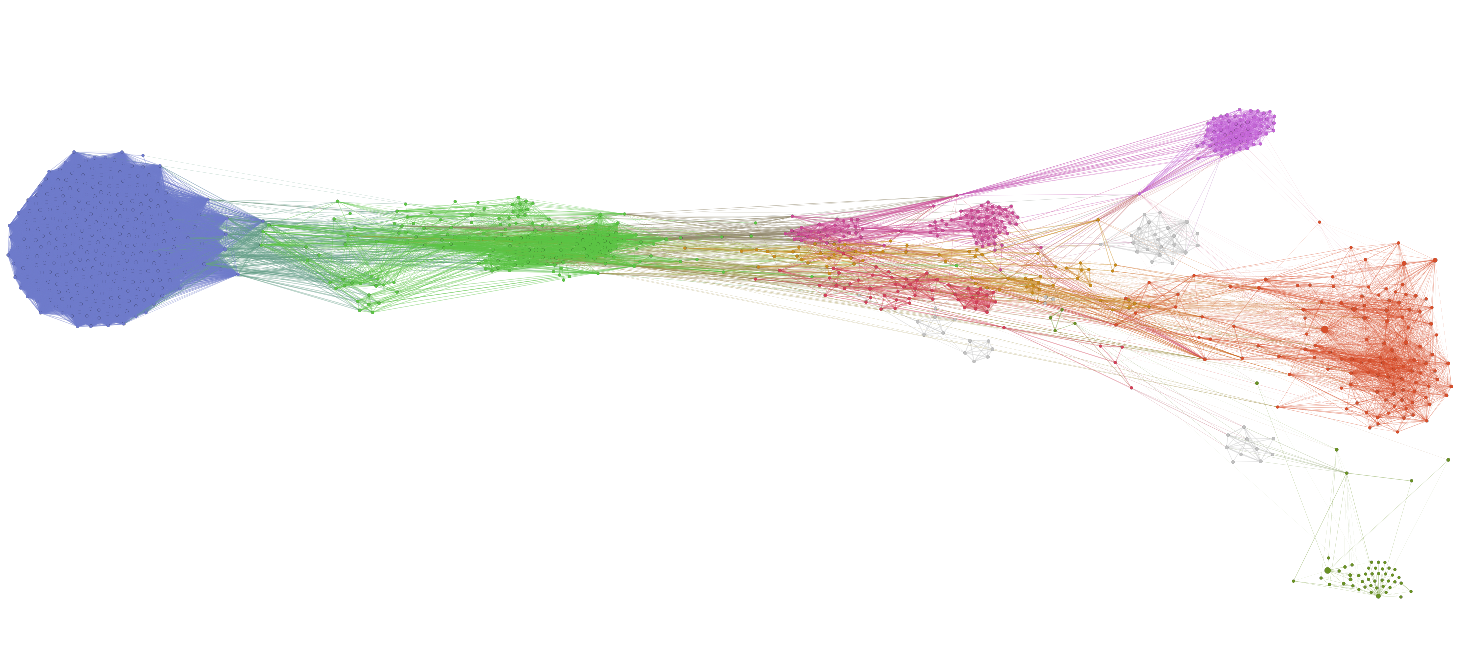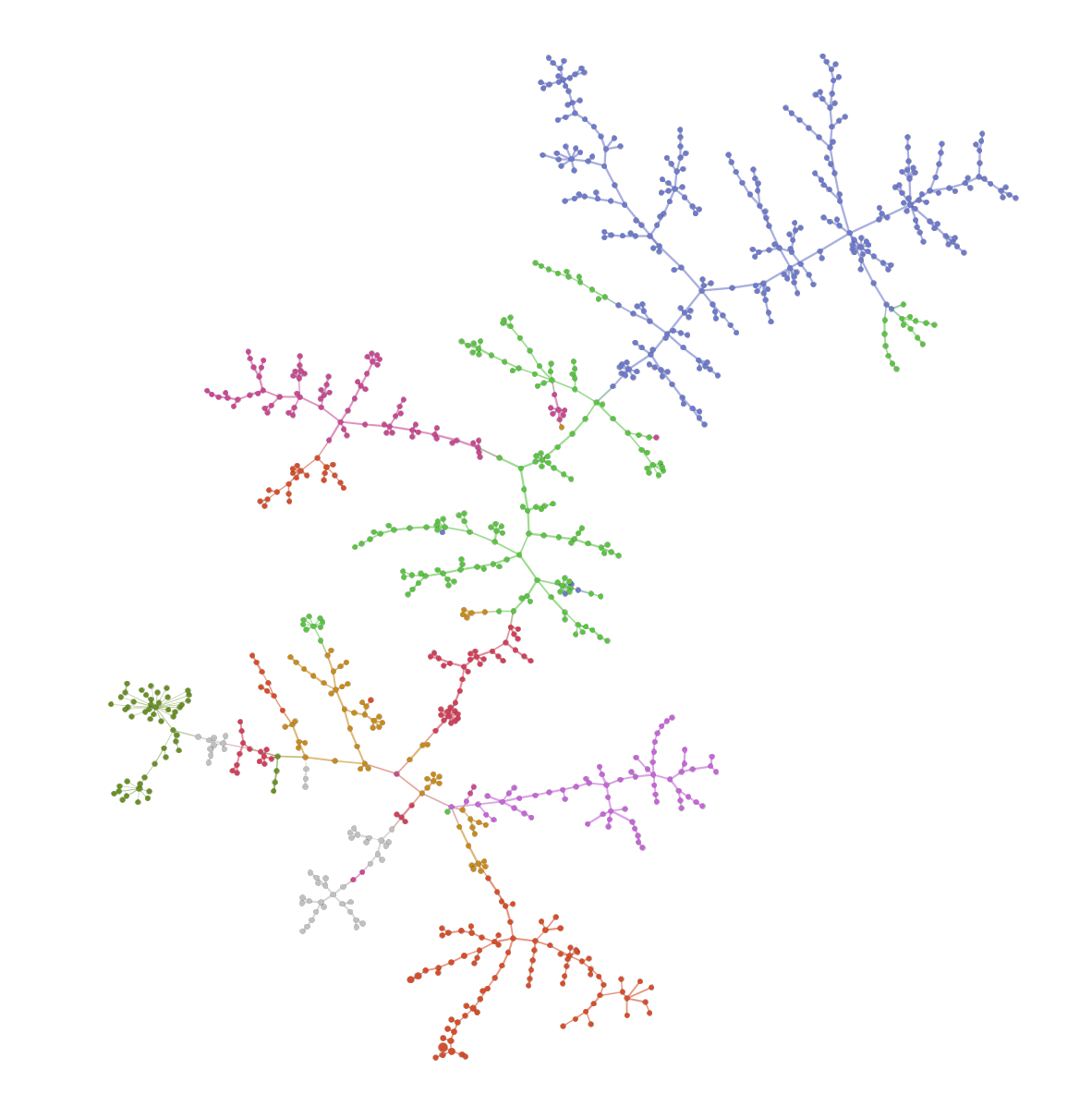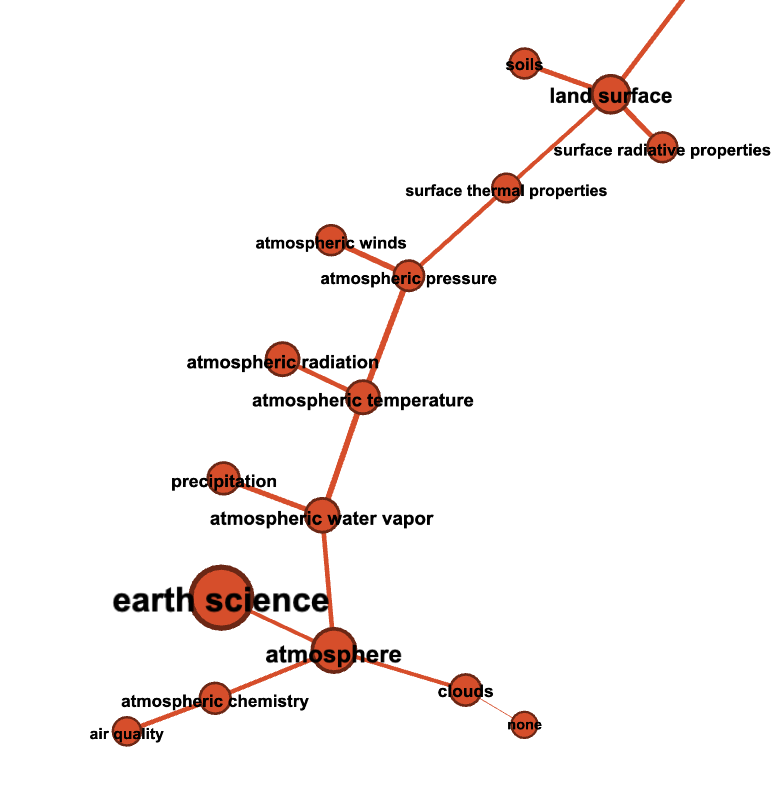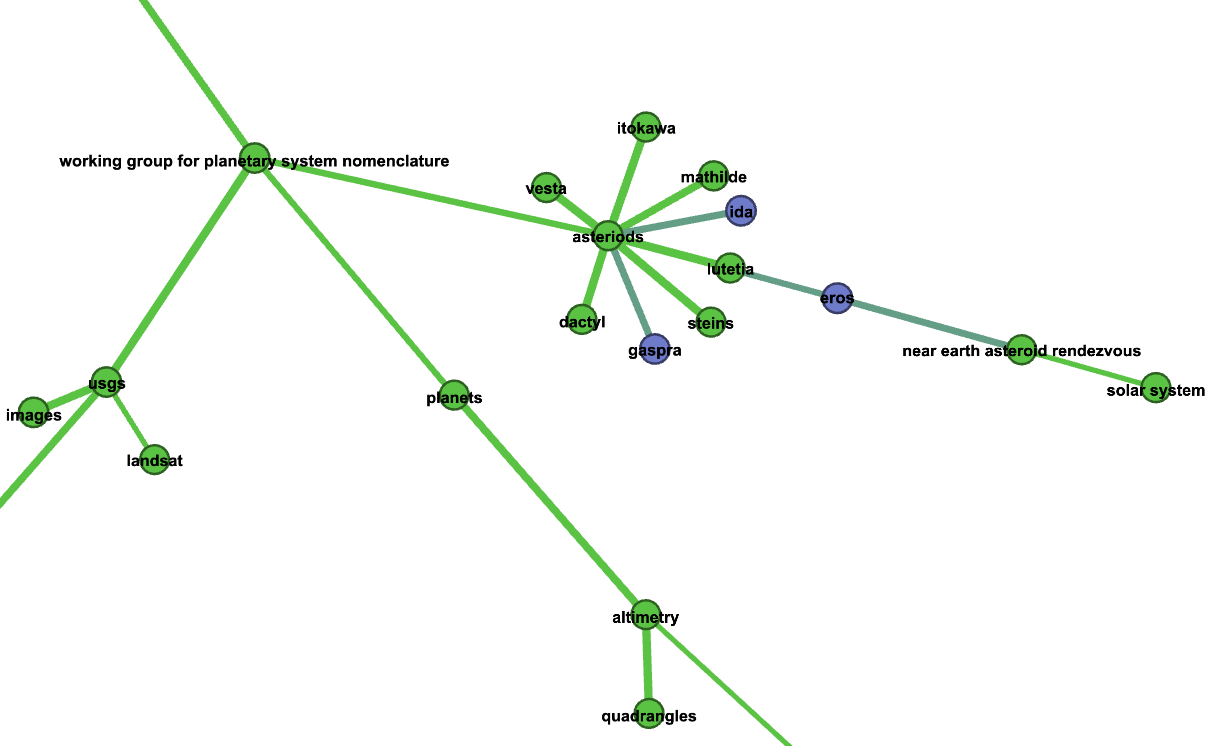# NASA dataset keywords analysis: Neo4j analytics tutorial¶

In this notebook we use graph-based co-occurrence analysis on the publicly available Data catalog of NASA (https://data.nasa.gov/browse, and the API endpoint https://data.nasa.gov/data.json). This dataset consists of the meta-data for different NASA datasets. The source notebook can be found here.

We will work on the sets of keywords attached to each dataset and build a keyword co-occurrence graph describing relations between different dataset keywords. The keyword relations in the above-mentioned graph are quantified using mutual-information-based scores (normalized pointwise mutual information).

See the related tutorial here: https://www.tidytextmining.com/nasa.html

In this tutorial we will use the Neo4j-based implementation of different analytics interfaces provided by BlueGraph. Therefore, in order to use it, you need a running instance of the Neo4j database (see installation instructions).

import os
import json
import pandas as pd
import requests
import getpass

from bluegraph.core import (PandasPGFrame,
pretty_print_paths,
pretty_print_tripaths)
from bluegraph.preprocess.generators import CooccurrenceGenerator
from bluegraph.backends.neo4j import (pgframe_to_neo4j,
Neo4jMetricProcessor,
Neo4jPathFinder,
Neo4jCommunityDetector,
Neo4jGraphProcessor)
from bluegraph.backends.networkx import NXPathFinder, networkx_to_pgframe


## Data preparation¶

NASA_META_DATA_URL = "https://data.nasa.gov/data.json"
if not os.path.isfile("../data/nasa.json"):
r = requests.get(NASA_META_DATA_URL)
open("../data/nasa.json", "wb").write(r.content)

with open("../data/nasa.json", "r") as f:

print("Example dataset: ")
print("----------------")
print(json.dumps(data["dataset"], indent="   "))

print()
print("Keywords: ", data["dataset"]["keyword"])

Example dataset:
----------------
{
"accessLevel": "public",
"landingPage": "https://pds.nasa.gov/ds-view/pds/viewDataset.jsp?dsid=RO-E-RPCMAG-2-EAR2-RAW-V3.0",
"bureauCode": [
"026:00"
],
"issued": "2018-06-26",
"@type": "dcat:Dataset",
"modified": "2020-03-04",
"references": [
"https://pds.nasa.gov"
],
"keyword": [
"earth",
"unknown",
"international rosetta mission"
],
"contactPoint": {
"@type": "vcard:Contact",
"fn": "Thomas Morgan",
"hasEmail": "mailto:thomas.h.morgan@nasa.gov"
},
"publisher": {
"@type": "org:Organization",
"name": "National Aeronautics and Space Administration"
},
"identifier": "urn:nasa:pds:context_pds3:data_set:data_set.ro-e-rpcmag-2-ear2-raw-v3.0",
"description": "This dataset contains EDITED RAW DATA of the second Earth Flyby (EAR2). The closest approach (CA) took place on November 13, 2007 at 20:57",
"title": "ROSETTA-ORBITER EARTH RPCMAG 2 EAR2 RAW V3.0",
"programCode": [
"026:005"
],
"distribution": [
{
"@type": "dcat:Distribution",
"mediaType": "text/html"
}
],
"accrualPeriodicity": "irregular",
"theme": [
"Earth Science"
]
}

Keywords:  ['earth', 'unknown', 'international rosetta mission']


Create a dataframe with keyword occurrence in different datasets

rows = []
for el in data['dataset']:
row = [el["identifier"]]
if "keyword" in el:
for k in el["keyword"]:
rows.append(row + [k])
keyword_data = pd.DataFrame(rows, columns=["dataset", "keyword"])

keyword_data

dataset keyword
0 urn:nasa:pds:context_pds3:data_set:data_set.ro... earth
1 urn:nasa:pds:context_pds3:data_set:data_set.ro... unknown
2 urn:nasa:pds:context_pds3:data_set:data_set.ro... international rosetta mission
3 TECHPORT_9532 completed
4 TECHPORT_9532 jet propulsion laboratory
... ... ...
112731 NASA-877__2 lunar
112732 NASA-877__2 jsc
112733 NASA-877__2 sample
112734 TECHPORT_94299 active
112735 TECHPORT_94299 trustees of the colorado school of mines

112736 rows × 2 columns

Aggregate dataset ids for each keyword and select the 500 most frequently used keywords.

n = 500

aggregated_datasets = keyword_data.groupby("keyword").aggregate(set)["dataset"]
most_frequent_keywords = list(aggregated_datasets.apply(len).nlargest(n).index)
most_frequent_keywords[:5]

['completed',
'earth science',
'atmosphere',
'national geospatial data asset',
'ngda']


Create a property graph object whose nodes are unique keywords.

graph = PandasPGFrame()
graph.add_node_types({n: "Keyword" for n in most_frequent_keywords})


Add sets of dataset ids as properties of our keyword nodes.

aggregated_datasets.index.name = "@id"

graph._nodes.sample(5)

@type dataset
@id
4 vesta Keyword {urn:nasa:pds:context_pds3:data_set:data_set.d...
jsc Keyword {NASA-872, NASA-877, NASA-873, NASA-871, NASA-...
paaliaq Keyword {urn:nasa:pds:context_pds3:data_set:data_set.c...
international halley watch Keyword {urn:nasa:pds:context_pds3:data_set:data_set.i...
active remote sensing Keyword {C1243149604-ASF, C1243162394-ASF, C1243197502...
n_datasets = len(keyword_data["dataset"].unique())
print("Total number of dataset: ", n_datasets)

Total number of dataset:  25722


## Co-occurrence graph generation¶

We create a co-occurrence graph using the 500 most frequent keywords: nodes are keywords and a pair of nodes is connected with an undirected edge if two corresponding keywords co-occur in at lease one dataset. Moreover, the edges are equipped with weights corresponding to:

• raw co-occurrence frequency

• normalized pointwise mutual information (NPMI)

• frequency- and mutual-information-based distances (1 / frequency, 1 / NPMI)

generator = CooccurrenceGenerator(graph)
comention_edges = generator.generate_from_nodes(
"dataset", total_factor_instances=n_datasets,
compute_statistics=["frequency", "npmi"])

Examining 124750 pairs of terms for co-occurrence...


Remove edges with zero NPMI

comention_edges = comention_edges[comention_edges["npmi"] > 0]


Compute the NPMI-based distance score

comention_edges.loc[:, "distance_npmi"] = comention_edges.loc[:, "npmi"].apply(lambda x: 1 / x)


Add generated edges to the property graph.

graph.remove_node_properties("dataset") # Remove datasets from node properties
graph._edges = comention_edges.drop(columns=["common_factors"])
graph._edge_prop_types = {
"frequency": "numeric",
"npmi": "numeric",
"distance_npmi": "numeric"
}

graph.edges(raw_frame=True).sample(5)

frequency npmi distance_npmi
@source_id @target_id
surface radiative properties natural hazards 3 0.022294 44.855423
mars global surveyor stardust 2 0.395268 2.529931
sample treatment protocol temperature 1 0.446902 2.237629

## Initializing Neo4j graph from a PGFrame¶

In this section we will populate a Neo4j database with the generated keyword co-occurrence property graph.

In the cells below provide the credentials for connecting to your instance of the Neo4j database.

NEO4J_URI = "bolt://localhost:7687"
NEO4J_USER = "neo4j"

NEO4J_PASSWORD = getpass.getpass()

········


Populate the Neo4j database with the nodes and edges of the generated property graph using pgframe_to_neo4j. We specify labels of nodes (Keyword) and edges (CoOccurs) to use for the new elements.

NODE_LABEL = "Keyword"
EDGE_LABEL = "CoOccurs"

# (!) If you run this cell multiple times, you may create nodes and edges of the graph
# multiple times, if you have already run the notebook, set the parameter pgframe to None
# this will prevent population of the Neo4j database with the generated graph, but will create
# the necessary Neo4jGraphView object.
graph_view = pgframe_to_neo4j(
pgframe=graph,  # None, if no population is required
node_label=NODE_LABEL, edge_label=EDGE_LABEL,
directed=False)

# # If you want to clear the database from created elements, run
# graph_view._clear()


## Nearest neighours by NPMI¶

In this section we will compute top 10 neighbors of the keywords ‘mars’ and ‘saturn’ by the highest NPMI.

To do so, we will use the top_neighbors method of the PathFinder interface provided by the BlueGraph. This interface allows us to search for top neighbors with the highest edge weight. In this example, we use Neo4j-based Neo4jPathFinder interface.

path_finder = Neo4jPathFinder.from_graph_object(graph_view)

path_finder.top_neighbors("mars", 10, weight="npmi")

{'mars exploration rover': 0.7734334910676389,
'phoenix': 0.6468063979421724,
'mars science laboratory': 0.6354738555723674,
'2001 mars odyssey': 0.5902693119742288,
'mars global surveyor': 0.5756873488729959,
'mars reconnaissance orbiter': 0.555421194053889,
'viking': 0.5490894121185264,
'mars pathfinder': 0.5223639673427369,
'mars express': 0.5153112375202485,
'phobos': 0.49283414887183974}

path_finder.top_neighbors("saturn", 10, weight="npmi")

{'iapetus': 0.7512958866945076,
'tethys': 0.750629376973449,
'mimas': 0.7481024499128304,
'phoebe': 0.7458314316016054,
'rhea': 0.7453385116030462,
'dione': 0.7425859139664013,
'cassini-huygens': 0.74217432172955,
'hyperion': 0.7346061630878281,
'janus': 0.7144193057581066}


## Graph metrics and node centrality measures¶

BlueGraph provides the MetricProcessor interface for computing various graph statistics. We will use Neo4j-based Neo4jMetricProcessor interface.

metrics = Neo4jMetricProcessor.from_graph_object(graph_view)

print("Density of the constructed network: ", metrics.density())

Density of the constructed network:  0.051334669338677356


### Node centralities¶

In this example we will compute the Degree and PageRank centralities only for the raw frequency, and the Betweenness centrality for the mutual-information-based scores. We will use methods provided by the MetricProcessor interface in the write mode, i.e. computed metrics will be written as node properties of the underlying graph object.

Degree centrality is given by the sum of weights of all incident edges of the given node and characterizes the importance of the node in the network in terms of its connectivity to other nodes (high degree = high connectivity).

metrics.degree_centrality("frequency", write=True, write_property="degree")


PageRank centrality is another measure that estimated the importance of the given node in the network. Roughly speaking it can be interpreted as the probablity that having landed on a random node in the network we will jump to the given node (here the edge weights are taken into account”).

https://en.wikipedia.org/wiki/PageRank

metrics.pagerank_centrality("frequency", write=True, write_property="pagerank")


We then compute the betweenness centrality based on the NPMI distances.

Betweenness centrality is a node importance measure that estimates how often a shortest path between a pair of nodes will pass through the given node.

metrics.betweenness_centrality("distance_npmi", write=True, write_property="betweenness")

/Users/oshurko/opt/anaconda3/envs/bluegraph/lib/python3.6/site-packages/bluegraph/backends/neo4j/analyse/metrics.py:111: MetricProcessingWarning: Weighted betweenness centrality for Neo4j graphs is not implemented: computing the unweighted version
MetricProcessor.MetricProcessingWarning)


Now, we will export this backend-specific graph object into a PGFrame.

new_graph = metrics.get_pgframe(node_prop_types=graph._node_prop_types, edge_prop_types=graph._edge_prop_types)

new_graph.nodes(raw_frame=True).sample(5)

degree betweenness pagerank
@id
delta 25.0 122.525847 0.948799
langley research center 2.0 2.832536 0.553508
population 25.0 49.157125 0.729864
tarvos 32.0 0.000000 0.923662
print("Top 10 nodes by degree")
for n in new_graph.nodes(raw_frame=True).nlargest(10, columns=["degree"]).index:
print("\t", n)

Top 10 nodes by degree
earth science
jupiter
earth
land surface
terrestrial hydrosphere
imagery
support archives
sun
atmosphere
surface water

print("Top 10 nodes by PageRank")
for n in new_graph.nodes(raw_frame=True).nlargest(10, columns=["pagerank"]).index:
print("\t", n)

Top 10 nodes by PageRank
active
earth science
completed
project
pds
earth
jupiter
imagery
data
moon

print("Top 10 nodes by betweenness")
for n in new_graph.nodes(raw_frame=True).nlargest(10, columns=["betweenness"]).index:
print("\t", n)

Top 10 nodes by betweenness
astronomy
imagery
goddard space flight center
active
topography
safety
time
images
temperature


## Community detection¶

Community detection methods partition the graph into clusters of densely connected nodes in a way that nodes in the same community are more connected between themselves relatively to the nodes in different communities. In this section we will illustrate the use of the CommunityDetector interface provided by BlueGraph for community detection and estimation of its quality using modularity, performance and coverange methods.

First, we create a Neo4j-based instance and use several different community detection strategies provided by Neo4j.

com_detector = Neo4jCommunityDetector.from_graph_object(graph_view)


### Louvain algorithm¶

partition = com_detector.detect_communities(
strategy="louvain", weight="npmi")

print("Modularity: ", com_detector.evaluate_parition(partition, metric="modularity", weight="npmi"))
print("Performance: ", com_detector.evaluate_parition(partition, metric="performance", weight="npmi"))
print("Coverage: ", com_detector.evaluate_parition(partition, metric="coverage", weight="npmi"))

Modularity:  0.8055352122880087
Performance:  0.9050420841683366
Coverage:  0.9223953224304512


### Label propagation¶

partition = com_detector.detect_communities(
strategy="lpa", weight="npmi")

print("Modularity: ", com_detector.evaluate_parition(partition, metric="modularity", weight="npmi"))
print("Performance: ", com_detector.evaluate_parition(partition, metric="performance", weight="npmi"))
print("Coverage: ", com_detector.evaluate_parition(partition, metric="coverage", weight="npmi"))

Modularity:  0.6599097374293331
Performance:  0.6372184368737475
Coverage:  0.9699235341510681


### Writing community partition as node properties¶

com_detector.detect_communities(
strategy="louvain", weight="npmi",
write=True, write_property="louvain_community")

new_graph = com_detector.get_pgframe(
node_prop_types=new_graph._node_prop_types,
edge_prop_types=new_graph._edge_prop_types)

new_graph.nodes(raw_frame=True).sample(5)

degree betweenness louvain_community pagerank
@id
sample collection 29.0 251.402898 330 1.130440
atmospheric chemistry 50.0 134.337209 319 1.304748
neptune 48.0 2524.723644 353 1.602773
coanda 8.0 0.000000 141 0.696748
phobos 49.0 868.444670 353 1.426603

## Export network and the computed metrics¶

Save graph as JSON

new_graph.export_json("../data/nasa_comention.json")


Save the graph for Gephi import.

new_graph.export_to_gephi(
"../data/gephi_nasa_comention",
node_attr_mapping = {
"degree": "Degree",
"pagerank": "PageRank",
"betweenness": "Betweenness",
"louvain_community": "Community"
},
edge_attr_mapping={
"npmi": "Weight"
})


The representation of the network saved above can be imported into Gephi for producing graph visualizations, as in the following example:

In the figures below colors represent communities detected using the raw frequency of the co-occurrence edges, node sizes are proportional to the PageRank of nodes and edge thickness to the NPMI values.We can zoom into some of the communities of keywords identified using the community detection method above

Celestial bodies

Earth science

Space programs and missions

## Minimum spanning tree¶

A minimum spanning tree of a network is given by a subset of edges that make the network connected ($$n - 1$$ edges connecting $$n$$ nodes). Its weighted version minimizes not only the number of edges included in the tree, but the total edge weight.

In the following example we compute a minimum spanning tree minimizing the NPMI-based distance weight of the network edges. We use the Neo4j-based implementation of the PathFinder interface.

path_finder.minimum_spanning_tree(distance="distance_npmi", write=True, write_edge_label="MSTEdge")

nx_path_finder = NXPathFinder(new_graph, directed=False)
tree = nx_path_finder.minimum_spanning_tree(distance="distance_npmi")

tree_pgframe = networkx_to_pgframe(
tree,
node_prop_types=new_graph._node_prop_types,
edge_prop_types=new_graph._edge_prop_types)

tree_pgframe.export_to_gephi(
"../data/gephi_nasa_spanning_tree",
node_attr_mapping = {
"degree": "Degree",
"pagerank": "PageRank",
"betweenness": "Betweenness",
"louvain_community": "Community"
},
edge_attr_mapping={
"npmi": "Weight"
})Zoom Earth ScienceZoom Asteroids